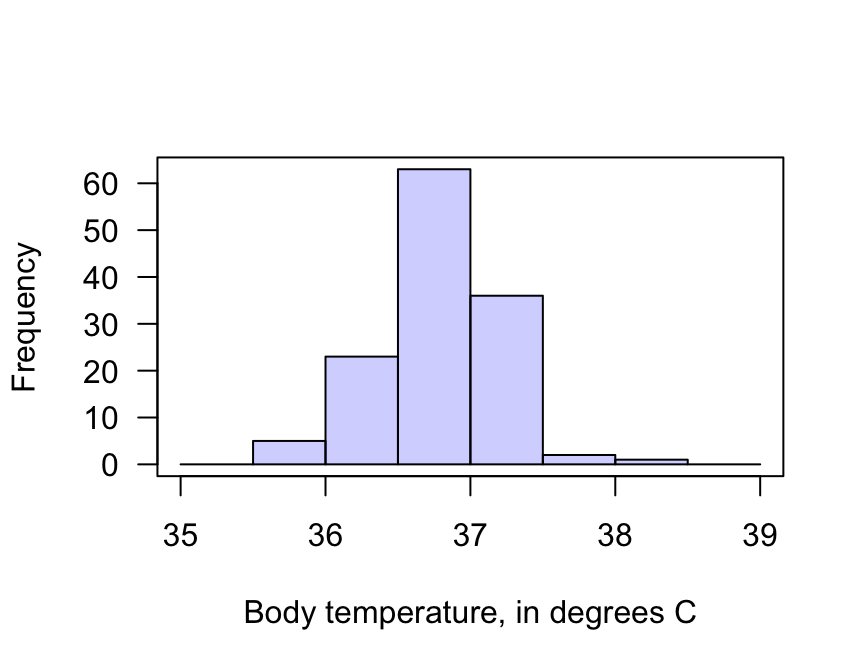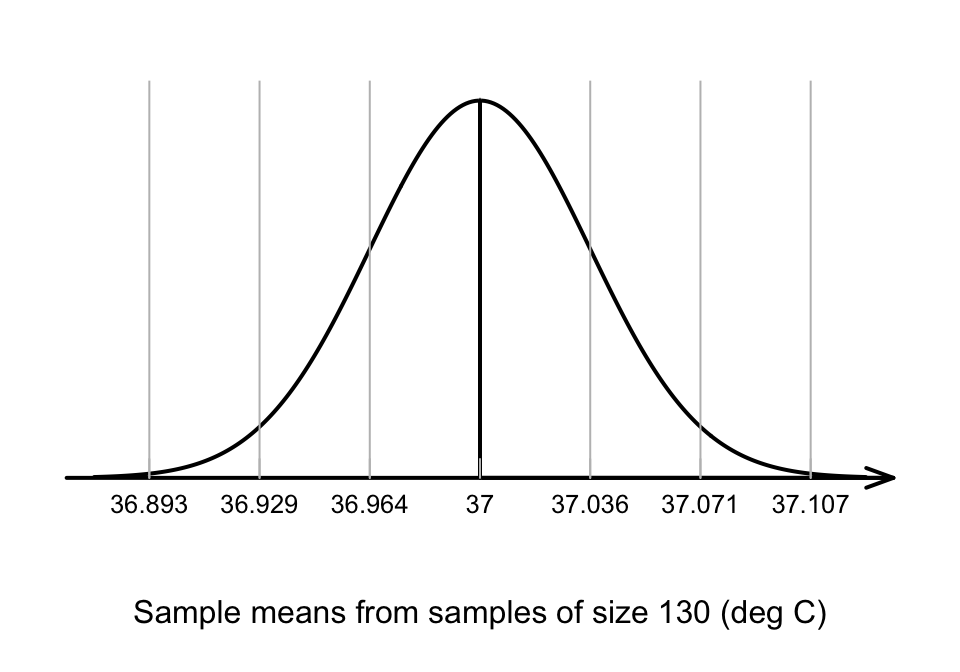## 27.3 Sampling distribution: One mean

A RQ is answered using data (this is partly what is meant by evidence-based research). Fortunately, for the body-temperature study, data are available from a comprehensive American study .

Summarising the data is important, because the data are the means by which the RQ is answered (data below).

A graphical summary (Fig. 27.1) shows that the internal body temperature of individuals varies from person to person: this is natural variation. A numerical summary (from software) shows that:

• The sample mean is $$\bar{x} = 36.8051^\circ$$C;
• The sample standard deviation is $$s = 0.40732^\circ$$C;
• The sample size is $$n=130$$.

The sample mean is less than the assumed value of $$\mu=37^\circ\text{C}$$… The question is why: can the difference reasonably be explained by sampling variation, or not?

A 95% CI can also be computed (using software or manually): the 95% CI for $$\mu$$ is from $$36.73^\circ$$ to $$36.88^\circ$$C. This CI is narrow, implying that $$\mu$$ has been estimated with precision, so detecting even small deviations of $$\mu$$ from $$37^\circ$$ should be possible.FIGURE 27.1: The histogram of the body temperature data

The decision-making process assumes that the population mean temperature is $$\mu=37.0^\circ\text{C}$$, as stated in the null hypothesis. Because of sampling variation, the value of $$\bar{x}$$ sometimes would be smaller than $$37.0^\circ\text{C}$$ and sometimes greater than $$37.0^\circ\text{C}$$.

How much variation in the value of $$\bar{x}$$ could be expected, simply due to sampling variation, when $$\mu=37.0^\circ\text{C}$$? This variation is described by the sampling distribution.

The sampling distribution of $$\bar{x}$$ was discussed in Sect. 22.2 (and Def. 22.1 specifically). From this, if $$\mu$$ really was $$37.0^\circ$$C and if certain conditions are true, the possible values of the sample means can be described using:

• An approximate normal distribution;
• With mean $$37.0^\circ\text{C}$$ (from $$H_0$$);
• With standard deviation of $$\displaystyle \text{s.e.}(\bar{x}) = \frac{s}{\sqrt{n}} = \frac{0.40732}{\sqrt{130}} = 0.035724$$. This is the standard error of the sample means.

A picture of this sampling distribution (Fig. 27.2) shows how the sample mean varies when $$n=130$$, simply due to sampling variation, when $$\mu = 37^\circ\text{C}$$. This enables questions to be asked about the likely values of $$\bar{x}$$ that would be found in the sample, when the population mean is $$\mu = 37^\circ\text{C}$$.FIGURE 27.2: The distribution of sample mean body temperatures, if the population mean is $$37^\circ$$C and $$n=130$$. The grey vertical lines are 1, 2 and 3 standard deviations from the mean.

Think 27.1 (Values of $$\bar{x}$$) Given the sampling distribution shown in Fig. 27.2, use the 68–95–99.7 rule to determine how often will $$\bar{x}$$ be larger than 37.036 degrees C just because of sampling variation, if $$\mu$$ really is $$37^\circ$$C.
About 16% of the time.

### References

Shoemaker AL. What’s normal? – temperature, gender, and heart rate. Journal of Statistics Education [Internet]. 1996;4(2). Available from: http://jse.amstat.org/v4n2/datasets.shoemaker.html.